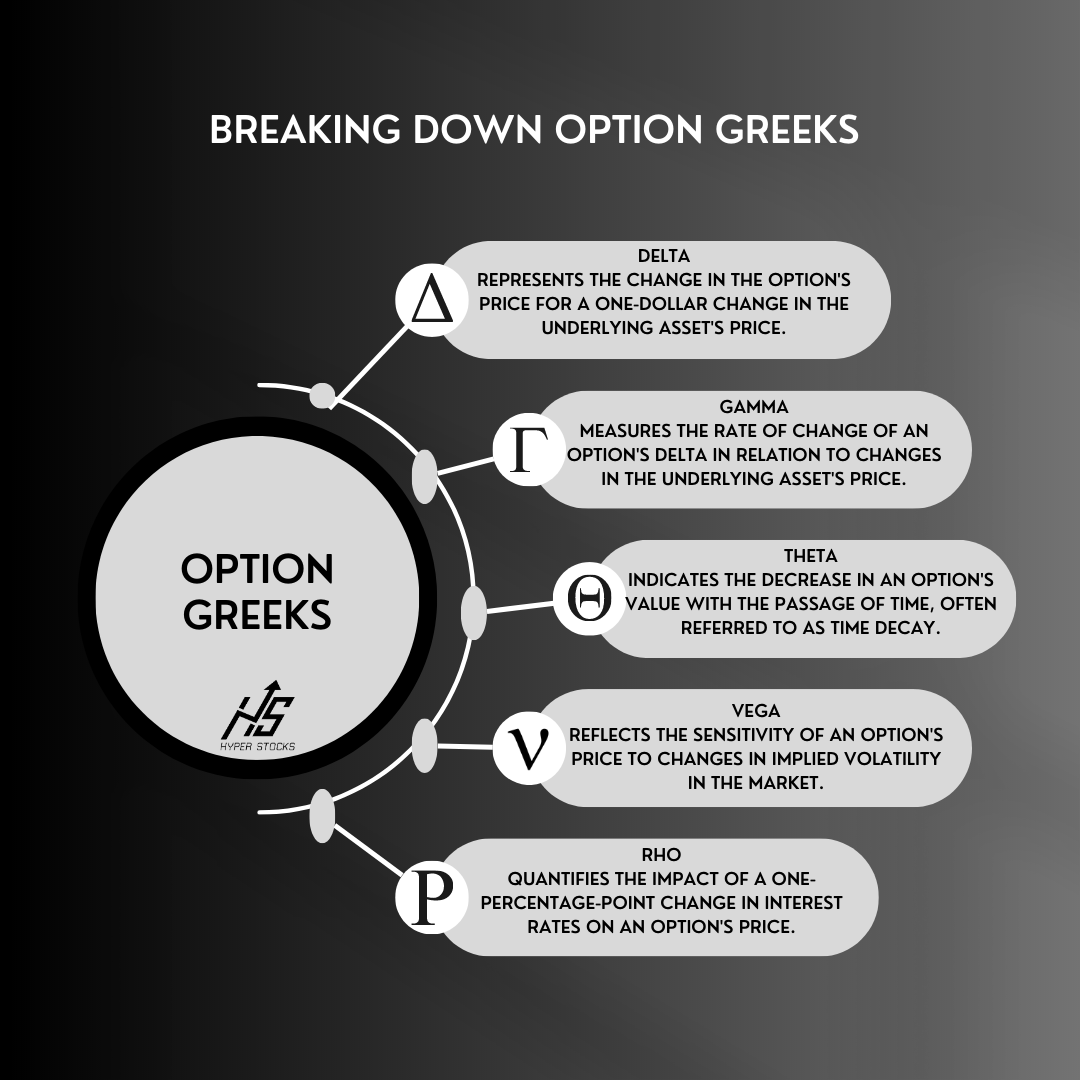# Option Greeks (Chart Cheat Sheet)To measure the value of change, multiply The Greek by 100.

For example:

If you're looking at an option contract and The Greek letter Delta is .50, then you multiple .50 by 100 to get the total amount you'll make or lose per dollar gain or loss on the underlying stock. In this case, Delta is .50, so if the underlying stock goes up by \$1.00 and you're in a call, then the option contract premium (value) will increase by about \$50.

Theta and time decay:

Theta is always a negative number because it represents time decay. Since option contracts have an expiration date, time is always working against the buyer, and it will starting working against the buyers faster as the expiration date approaches. If Theta is -.10, then the contract will lose about \$10 each day it is open assuming all other things remain equal.

### Primary Greeks:

1. Delta: Represents the change in the option's price for a one-point change in the underlying asset's price.
2. Gamma: Measures the rate of change of an option's delta in relation to changes in the underlying asset's price.
3. Theta: Indicates the decrease in an option's value with the passage of time, often referred to as time decay.
4. Vega: Reflects the sensitivity of an option's price to changes in implied volatility in the market.
5. Rho: Quantifies the impact of a one-percentage-point change in interest rates on an option's price.